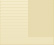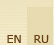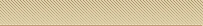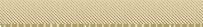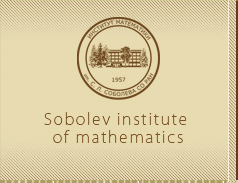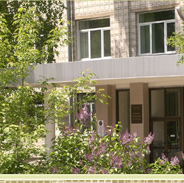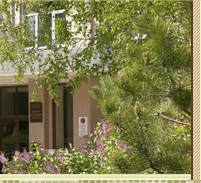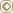MainWeb-postSite map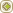Scientific subdivisions

 The name of the laboratory Head

 Laboratory of Algebra Kolesnikov P.S. Laboratory of Probability and Mathematical Statistics Lotov V.I. Laboratory of Fundamental Problems of Mathematics in Digital Technologies Volkov Y.S. Laboratory of Theoretical Physics Achasov N.N. International Mathematical Center Vdovin E.P. Laboratory Geometric Control Theory Vodop'yanov S.K. Laboratory of Functional Analysis Gutman A.E. Laboratory of Riemannian Geometry and Topology Bazaikin Y.V. Laboratory of Numerical Questions of Problems of Mathematical Physics Laboratory of Differential Equations and Close Points of Analysis Belonosov V.S. Laboratory of Differential and Difference Equations Demidenko G.V. Laboratory of Dynamical Systems Taimanov I.A. Laboratory of Data Analysis Laboratory of Discrete Analysis Evdokimov A.A. Discrete Optimization in Operations Research Pyatkin A.V. Laboratory "Mathematical Models of Decision Making" Beresnev V.L. Laboratory of Graph Theory Borodin O.V. Laboratory of algebraic combinatorics Avgustinovich S.V. Laboratory of Logical Systems Morozov A.S. Laboratory of Computation Theory and Applied Logic Laboratory of Ill-Posed Processes Anikonov D.S. Laboratory of Inverse Problems Mathematical Physics Neshchadim M.V. Laboratory of Function Theory Mednykh A.D. Laboratory of Numerical Methods of Mathematical Analysis Laboratory of Optimization Methods Bykadorov I.A.